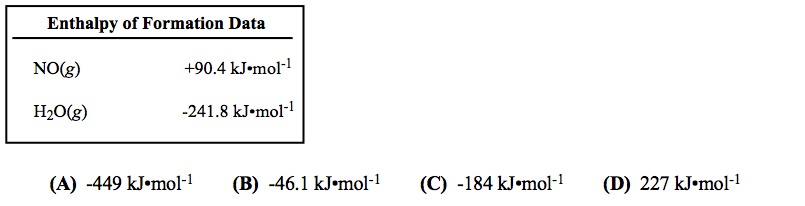# Problem: The combustion of ammonia is represented by this equation: 4NH3(g) + 5O2(g) → 4NO(g) + 6H2O(g)       ΔH°rxn = -904.8 kJ What is the enthalpy of formation of NH 3(g)?

###### FREE Expert Solution
84% (419 ratings)
###### Problem Details

The combustion of ammonia is represented by this equation:

4NH3(g) + 5O2(g) → 4NO(g) + 6H2O(g)       ΔH°rxn = -904.8 kJ

What is the enthalpy of formation of NH 3(g)?What scientific concept do you need to know in order to solve this problem?

Our tutors have indicated that to solve this problem you will need to apply the Enthalpy of Formation concept. You can view video lessons to learn Enthalpy of Formation. Or if you need more Enthalpy of Formation practice, you can also practice Enthalpy of Formation practice problems.

What is the difficulty of this problem?

Our tutors rated the difficulty ofThe combustion of ammonia is represented by this equation: ...as medium difficulty.

How long does this problem take to solve?

Our expert Chemistry tutor, Ellen took 3 minutes and 3 seconds to solve this problem. You can follow their steps in the video explanation above.

What professor is this problem relevant for?

Based on our data, we think this problem is relevant for Professor Arnold's class at IU.